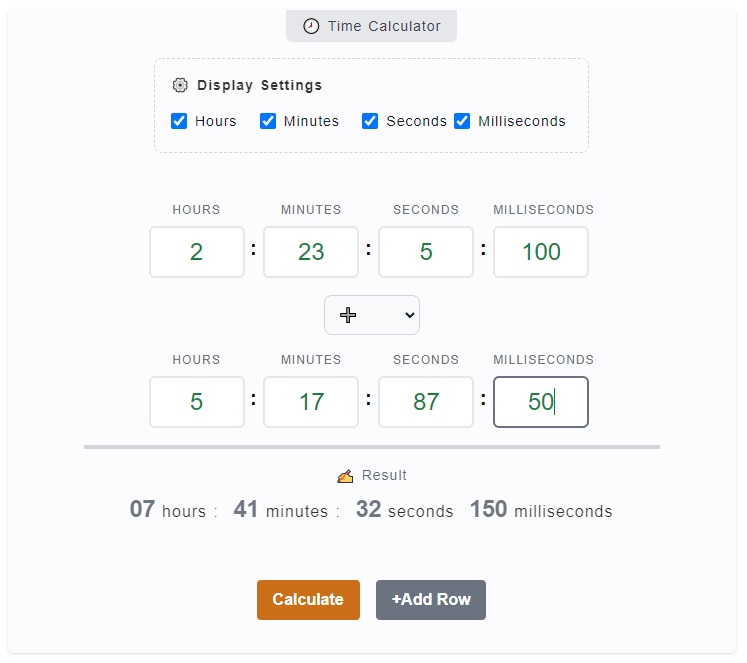# Time Calculator

The Time Calculator is used to perform four basic mathematical operations with time where you can add time, subtract time, multiply time and divide time. While we have a calculator for each of those operations, this is jam-packed with all of them. All you need is to change the operation according to your purpose and enter your time inputs.

🕗 Time Calculator

:
:
:
:
:
:

### ✍ Result

00 hours : 00 minutes :
00 seconds 00 milliseconds

## How to Use the Time Calculator

There are times that doing math on time is quite a hideous task, especially when you are dealing with odd numbers. How much more if there are multiple time entries. For instance, if you try to add `3 hours: 47 minutes`, `5 hours: 32 minutes`, `13 hours: 15 minutes`, it would probably take you some time to compute.

With this calculator, you can do all four (4) basic mathematical arithmetic operations with time. You can add, subtract, multiply and divide time. You just need to select your operation for that. Here's a quick guide to the calculator.1. Step 1

The first thing you might want to do is to check whether you need all hours, minutes, seconds and milliseconds input fields. You can omit the one's you don't need by just unchecking the corresponding checkbox on the `⚙️ Display Settings`.

2. Step 2

For the next step, there's this default empty set of time on the first row. It is marked with Hours, Minutes, Seconds and Milliseconds. Enter your base time in here and by that, what we mean is if you are adding time, it's called first addend. For subtraction it's where you add the minuend, multiplicand for multiplication or dividend for division.

3. Step 3

The third step is to select your mathematical operation. Below the first set of time inputs, there's a `select input field` where you can select the type of operation you want to perform. Choose one from addition (➕), subtraction (➖), multiplication (✖️) or division (➗).

4. Step 4

When you have picked the type of operation, you can now add the second set of time inputs or numbers. If you are performing addition or subtraction, you can enter the corresponding second addend or the subtrahend. They are both in time format. However, for multiplication and division, the multiplier and divisor are both in whole number format. You can play around with these inputs.

5. Step 4

After that, if there's a need for you to add another time input or number input, you can click on `+Add Row` button and you'll get another set of inputs. There's a `trash icon 🗑️` baside your added row in case you need to delete it. When all of this is done, you can click on `Calculate` button and the result will be displayed.

## Time Calculator Inputs and Outputs

This Time Calculator is built in a quite direct and simple approach. Using the calculator shouldn't be much of a work to do. However, let's take some time to know the inputs, options and results of the calculation.

### Display Settings

The display settings are added on the calculator for you to personalize your inputs. These are in the form of checkboxes, which is checked by default. It includes the `Hours`, `Minutes`, `Seconds` and `Milliseconds`. You can actually uncheck the one's you don't need and it will be hidden on the display.

### Inputs

The calculator has hours, minutes, seconds and milliseconds inputs. It is where you should enter the value that corresponds to the label. Don't mistaken that instead of 3 `hours`, you entered it on `minutes` input. For addition and subtraction, it's in time format but on multiplication and division, it's on number format.

### Operation Type

You can perform four mathematical operations in here - addition (➕), subtraction (➖), multiplication (✖️) and division (➗). You just need to click and select the one's you need.

### Outputs

The output or the result of the calculation is being displayed right below the input fields which is labeled with `✍ Result`. This serves as the results which could be your - sum, difference, product or quotient.

### Calculate Button

Make sure to click on the `Calculate` button every time you have finished entering your inputs. It will read all your input entries and operations and make the computation.

You have the option to add more rows where you can just simply click on `+Add Row` button. Then to remove it, just click on the `trash icon 🗑️`.